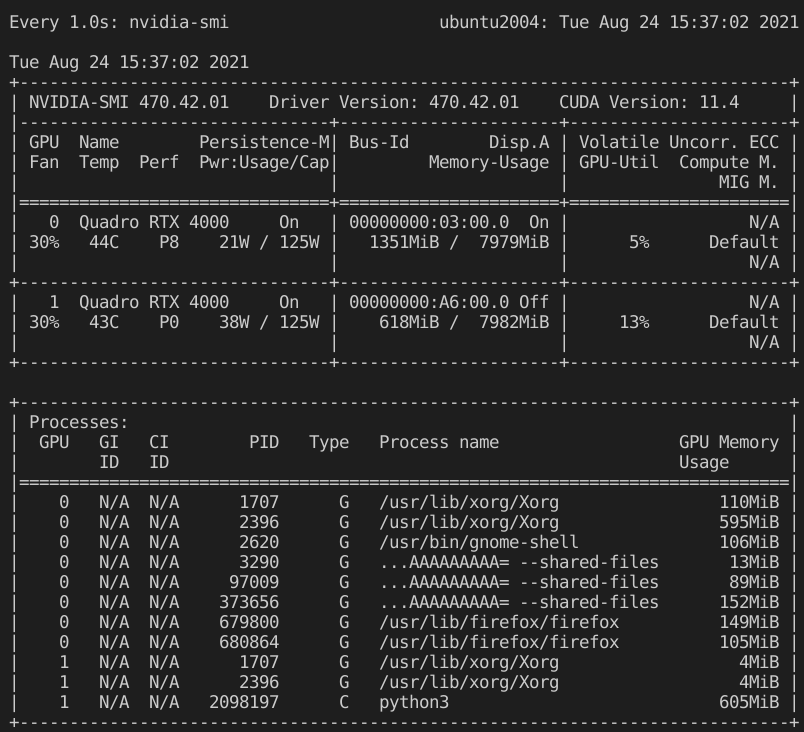技术背景

Numpy是在Python中非常常用的一个库，不仅具有良好的接口文档和生态，还具备了最顶级的性能，这个库很大程度上的弥补了Python本身性能上的缺陷。虽然我们也可以自己使用Cython或者是在Python中调用C++的动态链接库，但是我们自己实现的方法不一定有Numpy实现的快，这得益于Numpy对于SIMD等技术的深入实现，把CPU的性能发挥到了极致。因此我们只能考虑弯道超车，尝试下能否用自己实现的GPU的算法来打败Numpy的实现。

矩阵的元素乘

# cuda_test.py

import numpy as np
import time
from numba import cuda
cuda.select_device(1)

@cuda.jit
def CudaSquare(x):
i, j = cuda.grid(2)
x[i][j] *= x[i][j]

if __name__ == '__main__':
np.random.seed(1)
array_length = 2**10
random_array = np.random.rand(array_length, array_length)
random_array_cuda = cuda.to_device(random_array)
square_array = np.square(random_array)
CudaSquare[(array_length,array_length),(1,1)](random_array_cuda)
square_array_cuda = random_array_cuda.copy_to_host()
print (np.sum(square_array-square_array_cuda))

$python3 cuda_test.py 0.0 这个打印的结果表示，用numba的cuda方案与用numpy的square函数计算出来的结果差值是0，也就是得到了完全一样的结果。需要注意的是，在GPU上的向量是不能够直接打印出来的，需要先用copy_to_host的方法拷贝到CPU上再进行打印。 numba.cuda加速效果测试 在上一个测试案例中，为了展示结果的一致性，我们使用了内存拷贝的方法，但是实际上我们如果把所有的运算都放在GPU上面来运行的话，就不涉及到内存拷贝，因此这部分的时间在速度测试的过程中可以忽略不计。 # cuda_test.py import numpy as np import time from tqdm import trange from numba import cuda cuda.select_device(1) @cuda.jit def CudaSquare(x): i, j = cuda.grid(2) x[i][j] *= x[i][j] if __name__ == '__main__': numpy_time = 0 numba_time = 0 test_length = 1000 for i in trange(test_length): np.random.seed(i) array_length = 2**10 random_array = np.random.rand(array_length, array_length) random_array_cuda = cuda.to_device(random_array) time0 = time.time() square_array = np.square(random_array) time1 = time.time() CudaSquare[(array_length,array_length),(1,1)](random_array_cuda) time2 = time.time() numpy_time += time1-time0 numba_time += time2-time1 print ('The time cost of numpy is {}s for {} loops'.format(numpy_time, test_length)) print ('The time cost of numba is {}s for {} loops'.format(numba_time, test_length)) 在这个案例中，我们循环测试1000次的运行效果，测试对象是1024*1024大小的随机矩阵的平方算法。之所以需要这么多次数的测试，是因为numba的即时编译在第一次执行时会消耗一定的编译时间，但是编译完成后再调用，时间就会被大大的缩减。$ python3 cuda_test.py
100%|██████████████████████████████████████| 1000/1000 [00:13<00:00, 76.83it/s]
The time cost of numpy is 1.4523804187774658s for 1000 loops
The time cost of numba is 0.46444034576416016s for 1000 loops

# cuda_test.py

import numpy as np
import time
from tqdm import trange
from numba import cuda
cuda.select_device(1)

@cuda.jit
def CudaSquare(x):
i, j = cuda.grid(2)
x[i][j] *= x[i][j]

if __name__ == '__main__':
numpy_time = 0
numba_time = 0
test_length = 1000
for i in trange(test_length):
np.random.seed(i)
array_length = 2**12
random_array = np.random.rand(array_length, array_length)
random_array_cuda = cuda.to_device(random_array)
time0 = time.time()
square_array = np.square(random_array)
time1 = time.time()
CudaSquare[(array_length,array_length),(1,1)](random_array_cuda)
time2 = time.time()
numpy_time += time1-time0
numba_time += time2-time1
print ('The time cost of numpy is {}s for {} loops'.format(numpy_time, test_length))
print ('The time cost of numba is {}s for {} loops'.format(numba_time, test_length))

\$ python3 cuda_test.py
100%|████████████████████████████████████████| 100/100 [00:22<00:00,  4.40it/s]
The time cost of numpy is 4.878739595413208s for 100 loops
The time cost of numba is 0.3255774974822998s for 100 loops总结概要

Numpy这个库在Python编程中非常的常用，不仅在性能上补足了Python语言的一些固有缺陷，还具有无与伦比的强大生态。但是即使都是使用Python，Numpy也未必就达到了性能的巅峰，对于我们自己日常中使用到的一些计算的场景，针对性的使用CUDA的功能来进行GPU的优化，是可以达到比Numpy更高的性能的。

版权声明

“留一手”加剧内卷，“讲不清”浪费时间。
posted @ 2021-08-24 17:40  DECHIN  阅读(707)  评论(0编辑  收藏  举报